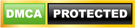Blog

# Condition Objects - Python

• ###### | 1596 Ratings

If you have knowledge of other programming languages, then you would know the importance of conditional statements. Conditional statements are required for taking decisions. Whenever we operate the software, our choice of action decides the next step. A simple example is booking an air ticket. If you want to travel in economy class, you will be presented a different package, whereas if you opt for business class you will be given a different package. So, let’s take a look at the conditional statements available in python.

Learn how to use Python, from beginner basics to advanced techniques, with online video tutorials taught by industry experts. Enroll for Free Python Training Demo!

-An else statement can be combined with an if statement. An else statement contains the block of code that executes if the conditional expression in the if statement resolves to 0 or a FALSE value.

Format:

```if (expression): code1

else:

code2```

EXAMPLE:

```a= 7
b=8
if(a>b):
print ‘I am greater than b’ else:
print ‘I am greater than a’```

Output :I am greater than a

-To check multiple conditions and run the block of code for the condition that returns ‘true’

Format:

```if expression1: code1

elif expression2: code2

elif expression3: code3

else:

code4```

EXAMPLE:

```a=7
b=7
if(a>b):
print ‘I am greater than b’ elif (b>a):
print ‘I am greater than a’ elif (a==b):
print ‘Hey!! we both are equal’```

Output: Hey!! we both are equal

- A simple if statement can be used without any else or else if. Usage of simple if statement means that the lines of code should be executed only if the condition is true.

Format:

`if condition : execute the code`

EXAMPLE:

```a=7
b=7
if(a>b):
print ‘I am greater than b’ print ‘Good Bye’```

Output: Good Bye